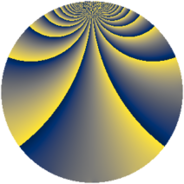# Properties

 Label 441.2.yLevel $441$ Weight $2$ Character orbit 441.y Rep. character $\chi_{441}(25,\cdot)$ Character field $\Q(\zeta_{21})$ Dimension $648$ Newform subspaces $1$ Sturm bound $112$ Trace bound $0$

# Related objects

## Defining parameters

 Level: $$N$$ $$=$$ $$441 = 3^{2} \cdot 7^{2}$$ Weight: $$k$$ $$=$$ $$2$$ Character orbit: $$[\chi]$$ $$=$$ 441.y (of order $$21$$ and degree $$12$$) Character conductor: $$\operatorname{cond}(\chi)$$ $$=$$ $$441$$ Character field: $$\Q(\zeta_{21})$$ Newform subspaces: $$1$$ Sturm bound: $$112$$ Trace bound: $$0$$

## Dimensions

The following table gives the dimensions of various subspaces of $$M_{2}(441, [\chi])$$.

Total New Old
Modular forms 696 696 0
Cusp forms 648 648 0
Eisenstein series 48 48 0

## Trace form

 $$648q - 5q^{2} - 13q^{3} - 109q^{4} - 12q^{5} + 2q^{6} - 7q^{7} - 16q^{8} - 3q^{9} + O(q^{10})$$ $$648q - 5q^{2} - 13q^{3} - 109q^{4} - 12q^{5} + 2q^{6} - 7q^{7} - 16q^{8} - 3q^{9} - 22q^{10} - 8q^{11} - 64q^{12} - 4q^{13} - 26q^{14} - 4q^{15} - 97q^{16} - 37q^{17} - q^{18} - 14q^{19} - 11q^{20} - 33q^{21} - q^{22} + 31q^{23} - 20q^{24} + 38q^{25} - 44q^{26} - 49q^{27} - 22q^{28} + 73q^{29} - 12q^{30} - 20q^{31} - q^{32} - 43q^{33} - 7q^{34} - 32q^{35} + 20q^{36} - 39q^{37} - 40q^{38} + q^{39} - q^{40} - 17q^{41} + 82q^{42} - q^{43} - 31q^{44} - 177q^{45} + 56q^{46} + 5q^{47} - 23q^{48} - q^{49} - 21q^{50} - 18q^{51} - 48q^{52} + 50q^{53} - 15q^{54} + 20q^{55} + 127q^{56} + 33q^{57} - 82q^{58} + 53q^{59} + 43q^{60} + 42q^{61} - 30q^{62} + 57q^{63} - 88q^{64} - 11q^{65} - 90q^{66} - 26q^{67} + 145q^{68} + 64q^{69} - 46q^{70} - 19q^{71} - 46q^{72} - 40q^{73} + 18q^{74} - 114q^{75} + 43q^{76} + 7q^{77} - 129q^{78} - 26q^{79} + 163q^{80} + 85q^{81} - 28q^{82} - 221q^{83} - 113q^{84} - 10q^{85} - 18q^{86} + 37q^{87} - 4q^{88} - 104q^{89} - 60q^{90} - 49q^{91} + 119q^{92} - 149q^{93} - 13q^{94} + 37q^{95} + 186q^{96} - 11q^{97} - 33q^{98} + 62q^{99} + O(q^{100})$$

## Decomposition of $$S_{2}^{\mathrm{new}}(441, [\chi])$$ into newform subspaces

Label Dim. $$A$$ Field CM Traces $q$-expansion
$$a_2$$ $$a_3$$ $$a_5$$ $$a_7$$
441.2.y.a $$648$$ $$3.521$$ None $$-5$$ $$-13$$ $$-12$$ $$-7$$